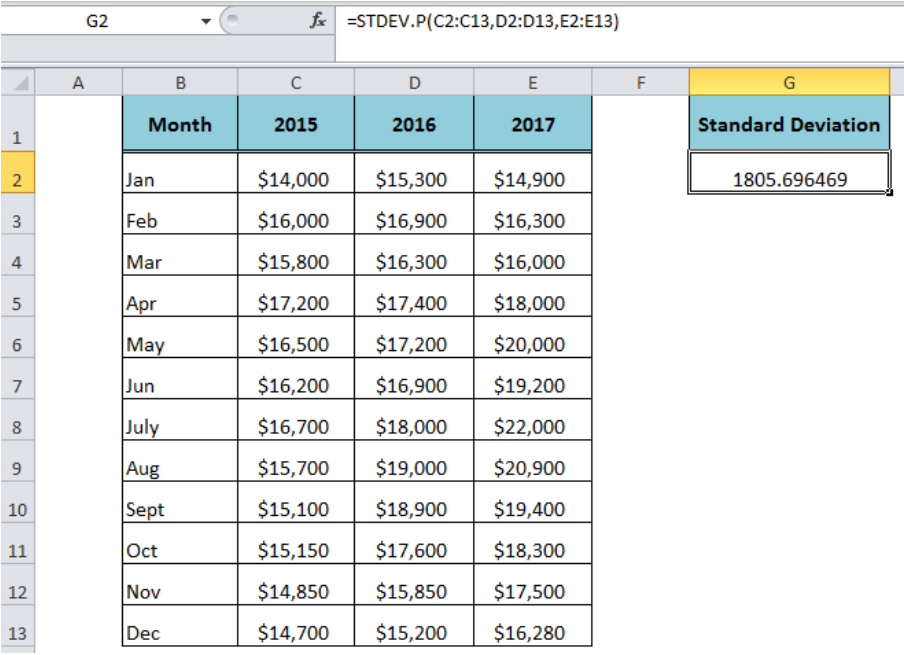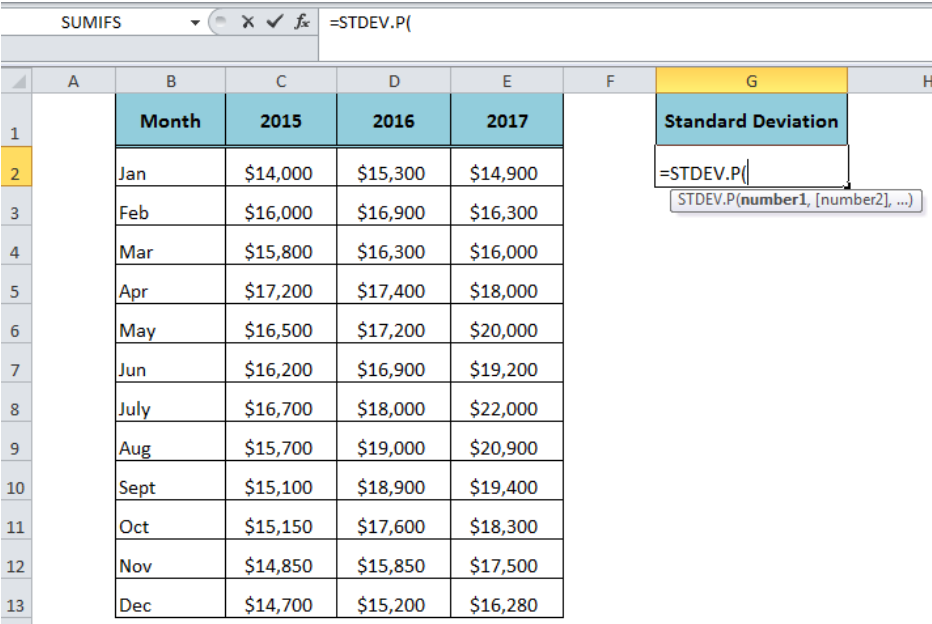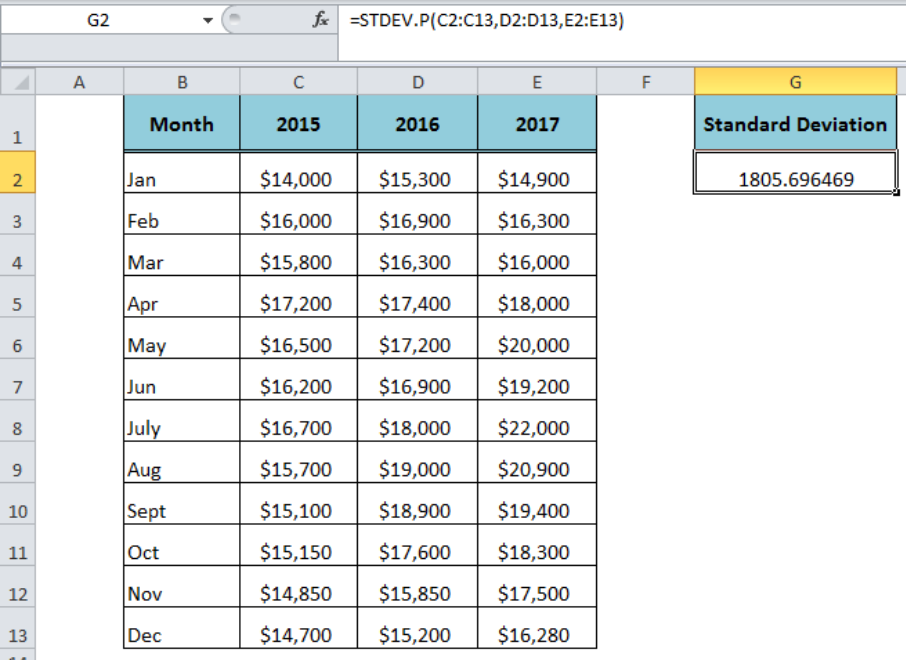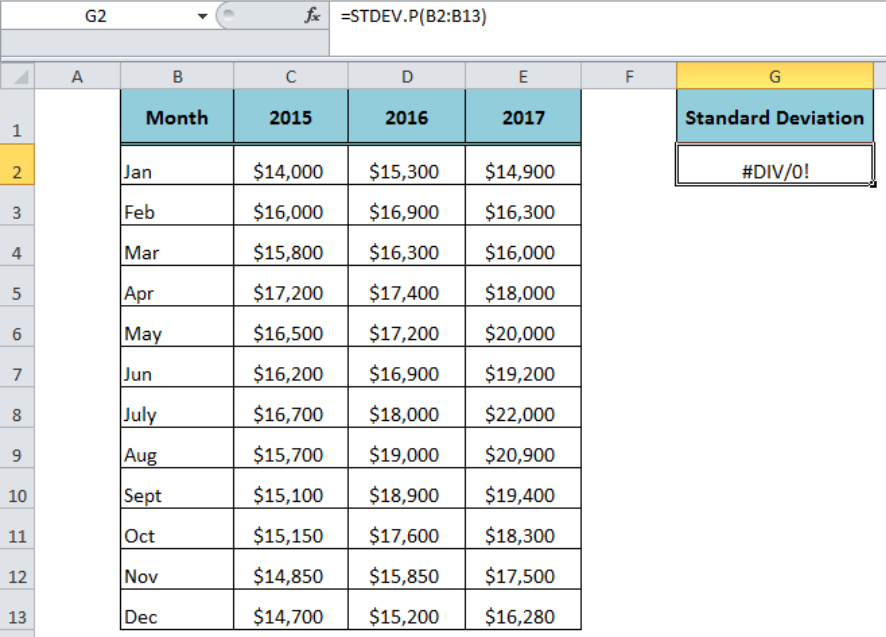Connect to our Experts within seconds for a free diagnosis
Our professional experts are available now. Your privacy is guaranteed.

# How to Use the Excel STDEV.P Function

The standard deviation is the statistical calculation of numeric dataset which measures how much widely spread the set of numbers are as compared to the average of the numbers. The Excel STDEV.P function returns the standard deviation of a population based on the entire population of numbers.Figure 1. How to Use Excel STDEV.P Function

## Syntax

The syntax for the Excel STDEV.P function is:

`=STDEV.P (number1, [number2], ... )`

Where,

The function has total 255 arguments.

• number1: It is required and the first argument of population number.
• [number2], ….): These are optional and between 2 to 255 arguments.

The arguments of the STDEV.P function can be numeric values, named ranges, arrays of values or cell references that may contain numbers, logical values, text values, empty cells, and text representations of numbers. It evaluates numeric values only, ignoring the text, logical value, empty cells and text representations of numbers available in cell references, ranges or arrays as arguments. However, logical values and text representations of numbers added directly as arguments in the STDEV.P function are evaluated as numbers.Figure 2. The Syntax For the STDEV.P Function

## Example

Suppose we have data set of last three years’ sales of a company and we need to calculate the standard deviation of the last three years sales data. As each year’s sales is available in a range of cells, so we need to supply three ranges in Excel STDEV.P function as arguments in the following formula;

`=STDEV.P(C2:C13,D2:D13,E2:E13)`Figure 3. The Output of the STDEV.P Function

## Usage Notes

• The STDEV.P function has been introduced in Excel 2010 to replace the STDEVP function. The STDEVP function is still available for compatibility with Excel 2007 and earlier versions.
• If none of the values are numeric in cell references, the function returns #DIV/0! error.
• If any of values supplied directly as an argument is a text value, it returns #VALUE! error.Figure 4. Error Value of the STDEV.P Function

## Instant Connection to an Expert through our Excelchat Service:

Most of the time, the problem you will need to solve will be more complex than a simple application of a formula or function. If you want to save hours of research and frustration, try our live Excelchat service! Our Excel Experts are available 24/7 to answer any Excel question you may have. We guarantee a connection within 30 seconds and a customized solution within 20 minutes.